Tom Kelliher, CS 240

Feb. 20, 2012

### From Last Time

Decoders, encoders, muxes.

### Outline

2. The lower bound for addition speed.

### Coming Up

1. Ripple carry adder: example of reuse and divide and conquer.
1. Wire together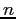full binary adders in order to add twobit numbers.

2. Running time of a ripple carry adder.

Running time of a full binary adder is O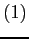. Ripple carry adder?

Excessive!!!

2. An O(!!!)bit adder.
1. Important equations (briefly explain):

Carry generate at bit position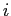: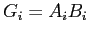.

Carry propagate at position: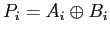.

2. Carry-in is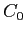.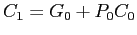.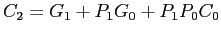.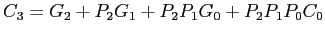.

Etc.

3. What's the circuit depth of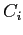?

4. What's wrong with this picture?

# The Lower Bound for Addition Speed

2. Our abstract gate for an idealized adder: thegate
1. Independent of technology.

2.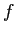is the fan-in.

3.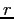is the radix (binary).

4. Assume that such a gate can compute any-valued function ofinputs.

3. Addingbit numbers. How many inputs? How many outputs? What is the minimum number of inputs some output is dependent upon? The maximum?

4. For the output dependent upon that maximum, consider the ideal circuit which reduces its inputs to the final output.
1. What does it look like?

2. How many intermediate signals remain after one level of gates?

3. What is its depth? -- That's the lower bound for addition.

Thomas P. Kelliher 2012-02-18
Tom Kelliher